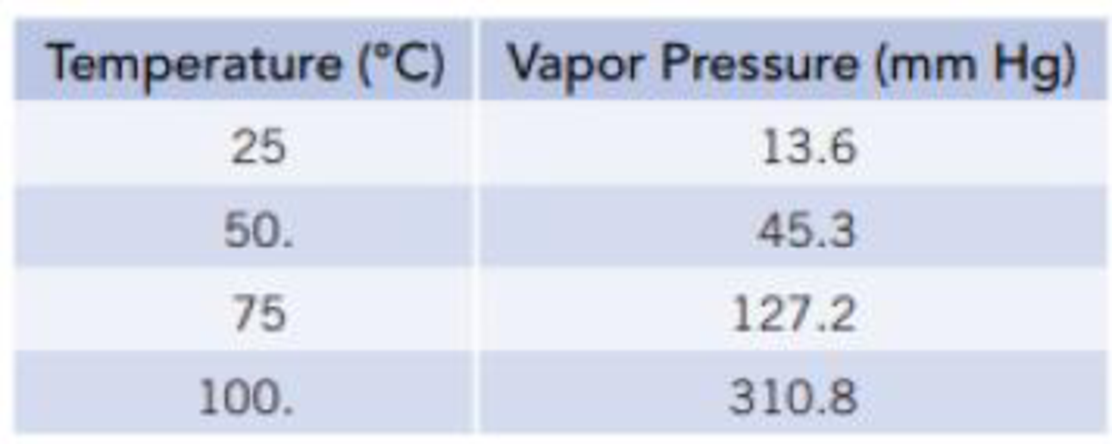Chapter 11, Problem 22PS

Chapter
Section
Textbook Problem

Vapor pressure data are given here for octane, C8H18.Use the Clausius–Clapeyron equation to calculate the molar enthalpy of vaporization of octane and its normal boiling point.

Interpretation Introduction

Interpretation:

The molar enthalpy of vaporization and boiling point of octane has to be determined.

Concept Introduction:

Clausius-Clapeyron equation:

lnP=(ΔvapH0RT)+C

From this relationship we can calculate the molar enthalpy of vaporization by knowing the corresponding temperature and pressure values.

If we have pressures at two different temperatures, then enthalpy of vaporization can be calculated by

lnP2p1=-ΔvapH0R[1T2-1T1]

Boiling point of a liquid: The temperature at which external pressure and vapour pressure of the liquid become same.

Normal boiling point: When the external pressure is 760mmHg we can call it as normal boiling point.

Explanation

Given:

Temperature(oC)Vapor Pressure(mmHg)2513.65045.375127.2100310.8

Temperatures and corresponding pressures are given. We can calculate the molar enthalpy of vaporization by using the Clausius-Clapeyron equation

lnP2p1=-ΔvapH0R[1T2-1T1]

• The molar enthalpy of vaporization is calculated using given data.

P1=13.6mmHg,P2=45.3mmHgT1=25°C=298K,T2=50°C=323K

Substituting the values

ln[45.313.6]=ΔvapH00.008314kJ/K.mol[1323K1298K]ln(1.2032)=ΔvapH00.008314kJ/K.mol[1323K1298K]ΔvapH0=38

Still sussing out bartleby?

Check out a sample textbook solution.

See a sample solution

The Solution to Your Study Problems

Bartleby provides explanations to thousands of textbook problems written by our experts, many with advanced degrees!

Get Started

Find more solutions based on key concepts